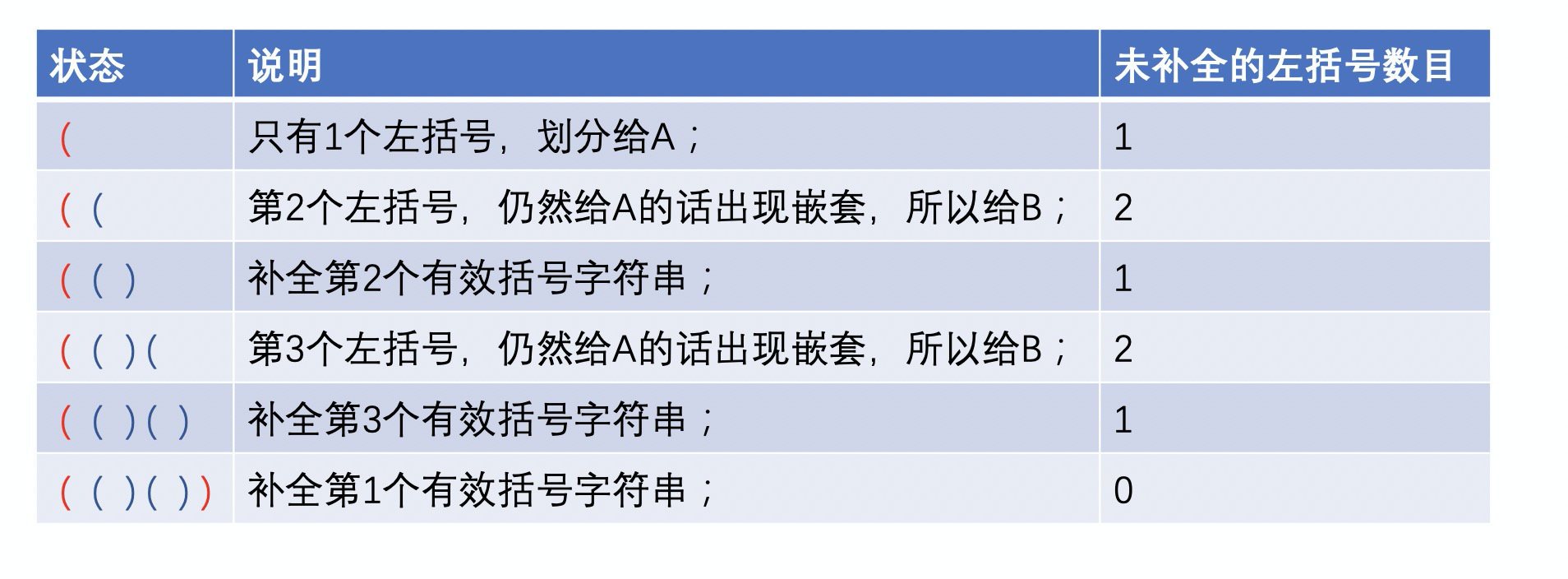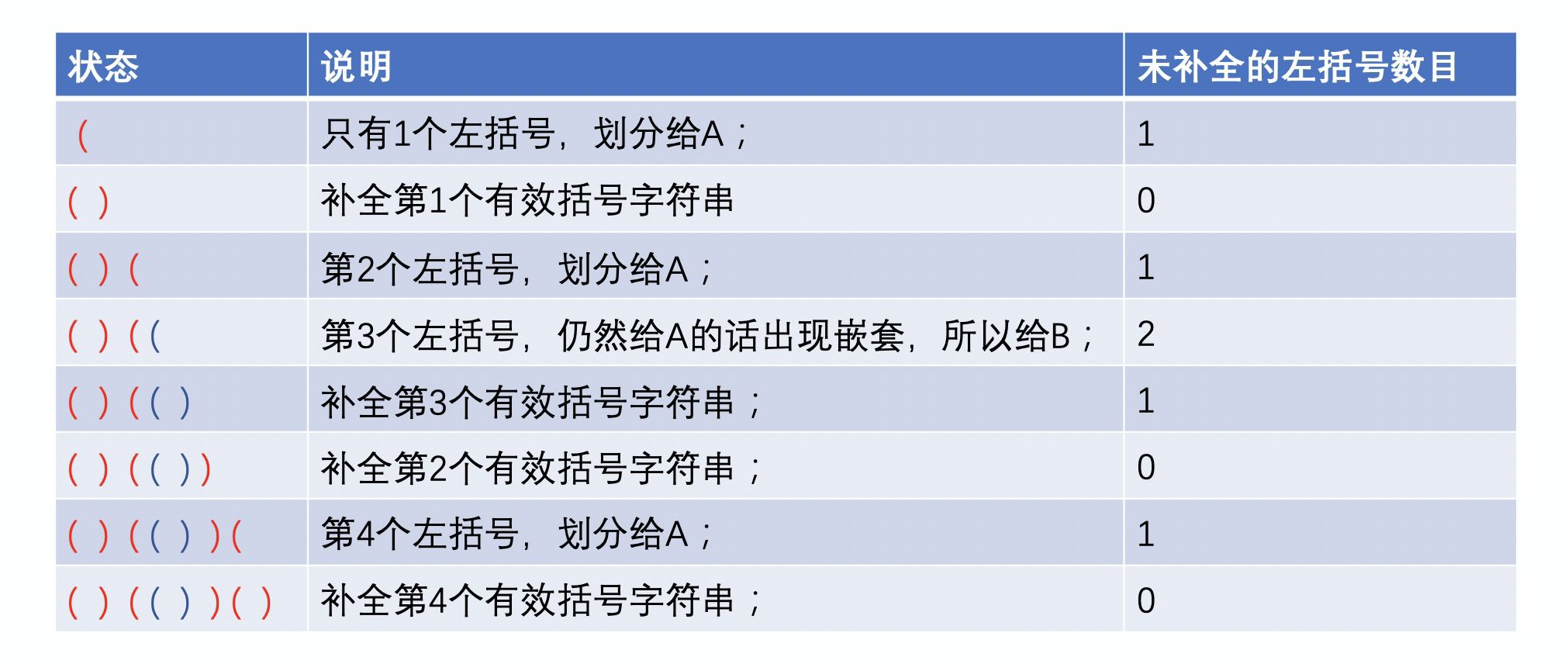@TOC

# # 题目描述

• 不相交：每个 `seq[i]` 只能分给 A 和 B 二者中的一个，不能既属于 A 也属于 B 。
• A 或 B 中的元素在原字符串中可以不连续。
• `A.length + B.length = seq.length`
• `max(depth(A), depth(B))` 的可能取值最小。

• `answer[i] = 0``seq[i]` 分给 A 。
• `answer[i] = 1``seq[i]` 分给 B 。 如果存在多个满足要求的答案，只需返回其中任意 一个 即可。

``````输入：seq = "(()())"

``````

``````输入：seq = "()(())()"

``````

1. `1 <= text.size <= 10000`

``````仅由 "(" 和 ")" 构成的字符串，对于每个左括号，都能找到与之对应的右括号，反之亦然。

1. 空字符串
2. 连接，可以记作 AB（A 与 B 连接），其中 A 和 B 都是有效括号字符串
3. 嵌套，可以记作 (A)，其中 A 是有效括号字符串

``````

``````  1. s 为空时，depth("") = 0
2. s 为 A 与 B 连接时，depth(A + B) = max(depth(A), depth(B))，其中 A 和 B 都是有效括号字符串
3. s 为嵌套情况，depth("(" + A + ")") = 1 + depth(A)，其中 A 是有效括号字符串

``````

# # 解题方法

1. 遇到左括号，如果未补全的左括号个数是奇数，把新的左括号给 A, 如果未补全的左括号个数是偶数，把新的左括号给 B。并且会让未补全的左括号数目加一；
2. 遇到右括号，右括号属于A或者B呢？和最后一个左括号分配给A或者B一致。并且会让未补全的左括号数目减一；

``````输入：seq = "(()())"

````````````输入：seq = "()(())()"

``````# # 代码

C++代码如下。

``````class Solution {
public:
vector<int> maxDepthAfterSplit(string seq) {
const int N = seq.size();
// 结果
vector<int> res(N, 0);
// 未补全的左括号数
int count = 0;
// 当前遍历到的结果的位置
int pos = 0;
// 遍历每个字符
for (char c : seq) {
if (c == '(') {
// 未补全的左括号数增加了
count ++;
// 如果未补全的左括号数是奇数，当前新的左括号分配给A，结果中写0；
// 如果未补全的左括号数是偶数，当前新的左括号分配给B，结果中写1；
res[pos] = 1 - count % 2;
} else {
// 右括号属于A或者B呢？和最后一个左括号分配给A或者B一致
res[pos] = 1 - count % 2;
// 未补全的左括号数减少了
count --;
}
// 结果的位置跟当前遍历到的字符相一致
pos ++;
}
return res;
}
};
``````# # 日期

2020 年 4 月 1 日 —— 纪念王伟烈士